# Mean State

Period Mean (original grids) [W/m2]
Model Period Mean (intersection) [W/m2]
Model Period Mean (complement) [W/m2]
Benchmark Period Mean (intersection) [W/m2]
Benchmark Period Mean (complement) [W/m2]
Bias [W/m2]
RMSE [W/m2]
Phase Shift [months]
Bias Score 
RMSE Score 
Seasonal Cycle Score 
Spatial Distribution Score 
Interannual Variability Score 
Overall Score 
Benchmark [-] 187.
CLM4 [-] 191. 191. 0.00 186. 206. 4.79 16.5 1.16 0.63 0.49 0.85 0.95 0.48 0.65
CLM4.5 [-] 192. 191. 0.00 186. 206. 5.03 16.6 1.18 0.62 0.49 0.85 0.96 0.48 0.65
CLM5 [-] 192. 192. 0.00 186. 206. 5.24 16.6 1.20 0.63 0.48 0.84 0.97 0.48 0.65
Period Mean (original grids) [W/m2]
Model Period Mean (intersection) [W/m2]
Model Period Mean (complement) [W/m2]
Benchmark Period Mean (intersection) [W/m2]
Benchmark Period Mean (complement) [W/m2]
Bias [W/m2]
RMSE [W/m2]
Phase Shift [months]
Bias Score 
RMSE Score 
Seasonal Cycle Score 
Spatial Distribution Score 
Interannual Variability Score 
Overall Score 
Benchmark [-] 198.
CLM4 [-] 196. 196. 0.00 198. 214. -1.20 18.5 1.30 0.57 0.48 0.82 0.93 0.74 0.67
CLM4.5 [-] 198. 198. 0.00 198. 214. -0.128 18.9 1.26 0.56 0.47 0.83 0.94 0.75 0.67
CLM5 [-] 198. 198. 0.00 198. 214. 0.401 18.8 1.49 0.57 0.47 0.78 0.95 0.74 0.66
Period Mean (original grids) [W/m2]
Model Period Mean (intersection) [W/m2]
Model Period Mean (complement) [W/m2]
Benchmark Period Mean (intersection) [W/m2]
Benchmark Period Mean (complement) [W/m2]
Bias [W/m2]
RMSE [W/m2]
Phase Shift [months]
Bias Score 
RMSE Score 
Seasonal Cycle Score 
Spatial Distribution Score 
Interannual Variability Score 
Overall Score 
Benchmark [-] 199.
CLM4 [-] 194. 194. 0.00 198. 219. -4.18 17.6 1.15 0.70 0.55 0.83 0.85 0.54 0.67
CLM4.5 [-] 195. 195. 0.00 198. 219. -3.73 17.5 1.16 0.70 0.55 0.83 0.84 0.54 0.67
CLM5 [-] 195. 195. 0.00 198. 219. -3.72 17.4 1.18 0.71 0.55 0.83 0.87 0.53 0.67
Period Mean (original grids) [W/m2]
Model Period Mean (intersection) [W/m2]
Model Period Mean (complement) [W/m2]
Benchmark Period Mean (intersection) [W/m2]
Benchmark Period Mean (complement) [W/m2]
Bias [W/m2]
RMSE [W/m2]
Phase Shift [months]
Bias Score 
RMSE Score 
Seasonal Cycle Score 
Spatial Distribution Score 
Interannual Variability Score 
Overall Score 
Benchmark [-] 70.7
CLM4 [-] 67.3 67.4 0.00 70.5 72.8 -3.59 24.0 0.468 0.90 0.71 0.97 1.0 0.73 0.83
CLM4.5 [-] 73.2 73.3 0.00 70.5 72.8 1.13 21.6 0.417 0.89 0.74 0.97 0.95 0.69 0.83
CLM5 [-] 75.8 75.9 0.00 70.5 72.8 3.73 21.2 0.340 0.87 0.76 0.98 0.97 0.68 0.83
Period Mean (original grids) [W/m2]
Model Period Mean (intersection) [W/m2]
Model Period Mean (complement) [W/m2]
Benchmark Period Mean (intersection) [W/m2]
Benchmark Period Mean (complement) [W/m2]
Bias [W/m2]
RMSE [W/m2]
Phase Shift [months]
Bias Score 
RMSE Score 
Seasonal Cycle Score 
Spatial Distribution Score 
Interannual Variability Score 
Overall Score 
Benchmark [-] 194.
CLM4 [-] 187. 186. 0.00 190. 207. -1.23 18.1 1.45 0.61 0.44 0.78 0.84 0.52 0.61
CLM4.5 [-] 187. 186. 0.00 190. 207. -1.29 18.1 1.48 0.61 0.44 0.77 0.84 0.52 0.60
CLM5 [-] 186. 186. 0.00 190. 207. -1.72 18.0 1.47 0.61 0.44 0.78 0.84 0.52 0.61
Period Mean (original grids) [W/m2]
Model Period Mean (intersection) [W/m2]
Model Period Mean (complement) [W/m2]
Benchmark Period Mean (intersection) [W/m2]
Benchmark Period Mean (complement) [W/m2]
Bias [W/m2]
RMSE [W/m2]
Phase Shift [months]
Bias Score 
RMSE Score 
Seasonal Cycle Score 
Spatial Distribution Score 
Interannual Variability Score 
Overall Score 
Benchmark [-] 97.8
CLM4 [-] 108. 108. 0.00 97.6 118. 10.3 21.4 0.265 0.85 0.79 0.98 0.98 0.73 0.85
CLM4.5 [-] 106. 106. 0.00 97.6 118. 8.50 23.0 0.274 0.84 0.77 0.98 0.95 0.74 0.84
CLM5 [-] 108. 108. 0.00 97.6 118. 11.0 20.9 0.266 0.85 0.79 0.98 1.0 0.72 0.86
Period Mean (original grids) [W/m2]
Model Period Mean (intersection) [W/m2]
Model Period Mean (complement) [W/m2]
Benchmark Period Mean (intersection) [W/m2]
Benchmark Period Mean (complement) [W/m2]
Bias [W/m2]
RMSE [W/m2]
Phase Shift [months]
Bias Score 
RMSE Score 
Seasonal Cycle Score 
Spatial Distribution Score 
Interannual Variability Score 
Overall Score 
Benchmark [-] 139.
CLM4 [-] 148. 148. 0.00 138. 149. 9.50 23.4 0.439 0.75 0.75 0.96 0.97 0.72 0.82
CLM4.5 [-] 147. 147. 0.00 138. 149. 8.85 23.2 0.441 0.76 0.75 0.96 0.98 0.72 0.82
CLM5 [-] 149. 149. 0.00 138. 149. 10.6 23.3 0.448 0.76 0.75 0.96 0.97 0.72 0.82
Period Mean (original grids) [W/m2]
Model Period Mean (intersection) [W/m2]
Model Period Mean (complement) [W/m2]
Benchmark Period Mean (intersection) [W/m2]
Benchmark Period Mean (complement) [W/m2]
Bias [W/m2]
RMSE [W/m2]
Phase Shift [months]
Bias Score 
RMSE Score 
Seasonal Cycle Score 
Spatial Distribution Score 
Interannual Variability Score 
Overall Score 
Benchmark [-] 181.
CLM4 [-] 192. 192. 0.00 179. 205. 12.5 25.1 0.341 0.59 0.58 0.97 0.99 0.66 0.73
CLM4.5 [-] 192. 193. 0.00 179. 205. 13.1 25.4 0.343 0.58 0.58 0.97 0.98 0.66 0.73
CLM5 [-] 193. 193. 0.00 179. 205. 13.7 25.4 0.335 0.58 0.59 0.97 0.96 0.65 0.72
Period Mean (original grids) [W/m2]
Model Period Mean (intersection) [W/m2]
Model Period Mean (complement) [W/m2]
Benchmark Period Mean (intersection) [W/m2]
Benchmark Period Mean (complement) [W/m2]
Bias [W/m2]
RMSE [W/m2]
Phase Shift [months]
Bias Score 
RMSE Score 
Seasonal Cycle Score 
Spatial Distribution Score 
Interannual Variability Score 
Overall Score 
Benchmark [-] 62.8
CLM4 [-] 62.2 62.7 0.00 63.2 54.4 -1.41 22.5 0.380 0.91 0.71 0.97 0.97 0.77 0.84
CLM4.5 [-] 63.9 64.4 0.00 63.2 54.4 -0.159 22.7 0.360 0.89 0.72 0.98 0.90 0.74 0.82
CLM5 [-] 68.8 69.2 0.00 63.2 54.4 4.51 19.9 0.302 0.89 0.76 0.98 0.94 0.72 0.84
Period Mean (original grids) [W/m2]
Model Period Mean (intersection) [W/m2]
Model Period Mean (complement) [W/m2]
Benchmark Period Mean (intersection) [W/m2]
Benchmark Period Mean (complement) [W/m2]
Bias [W/m2]
RMSE [W/m2]
Phase Shift [months]
Bias Score 
RMSE Score 
Seasonal Cycle Score 
Spatial Distribution Score 
Interannual Variability Score 
Overall Score 
Benchmark [-] 198.
CLM4 [-] 195. 195. 0.00 198. 197. -5.04 14.6 0.502 0.84 0.78 0.97 0.97 0.67 0.83
CLM4.5 [-] 195. 195. 0.00 198. 197. -5.00 14.7 0.491 0.84 0.78 0.97 0.97 0.67 0.83
CLM5 [-] 196. 196. 0.00 198. 197. -4.02 14.4 0.511 0.85 0.78 0.97 0.98 0.66 0.83
Period Mean (original grids) [W/m2]
Model Period Mean (intersection) [W/m2]
Model Period Mean (complement) [W/m2]
Benchmark Period Mean (intersection) [W/m2]
Benchmark Period Mean (complement) [W/m2]
Bias [W/m2]
RMSE [W/m2]
Phase Shift [months]
Bias Score 
RMSE Score 
Seasonal Cycle Score 
Spatial Distribution Score 
Interannual Variability Score 
Overall Score 
Benchmark [-] 120.
CLM4 [-] 131. 131. 0.00 118. 145. 13.8 25.1 0.251 0.78 0.79 0.98 1.0 0.75 0.85
CLM4.5 [-] 132. 132. 0.00 118. 145. 14.6 24.6 0.221 0.77 0.80 0.99 0.99 0.74 0.85
CLM5 [-] 132. 132. 0.00 118. 145. 14.6 24.3 0.228 0.77 0.80 0.98 0.99 0.73 0.85
Period Mean (original grids) [W/m2]
Model Period Mean (intersection) [W/m2]
Model Period Mean (complement) [W/m2]
Benchmark Period Mean (intersection) [W/m2]
Benchmark Period Mean (complement) [W/m2]
Bias [W/m2]
RMSE [W/m2]
Phase Shift [months]
Bias Score 
RMSE Score 
Seasonal Cycle Score 
Spatial Distribution Score 
Interannual Variability Score 
Overall Score 
Benchmark [-] 163.
CLM4 [-] 142. 142. 0.00 142. 173. 0.466 20.2 0.572 0.74 0.68 0.94 0.99 0.62 0.78
CLM4.5 [-] 142. 142. 0.00 142. 173. 0.983 19.8 0.577 0.75 0.69 0.94 1.0 0.62 0.78
CLM5 [-] 144. 144. 0.00 142. 173. 1.68 19.7 0.586 0.75 0.69 0.93 0.99 0.61 0.78
Period Mean (original grids) [W/m2]
Model Period Mean (intersection) [W/m2]
Model Period Mean (complement) [W/m2]
Benchmark Period Mean (intersection) [W/m2]
Benchmark Period Mean (complement) [W/m2]
Bias [W/m2]
RMSE [W/m2]
Phase Shift [months]
Bias Score 
RMSE Score 
Seasonal Cycle Score 
Spatial Distribution Score 
Interannual Variability Score 
Overall Score 
Benchmark [-] 177.
CLM4 [-] 166. 166. 0.00 175. 217. -8.23 18.4 0.379 0.71 0.75 0.97 0.98 0.69 0.81
CLM4.5 [-] 167. 167. 0.00 175. 217. -7.55 18.1 0.384 0.71 0.75 0.97 0.98 0.69 0.81
CLM5 [-] 168. 168. 0.00 175. 217. -6.31 17.8 0.410 0.72 0.75 0.96 0.98 0.71 0.81
Period Mean (original grids) [W/m2]
Model Period Mean (intersection) [W/m2]
Model Period Mean (complement) [W/m2]
Benchmark Period Mean (intersection) [W/m2]
Benchmark Period Mean (complement) [W/m2]
Bias [W/m2]
RMSE [W/m2]
Phase Shift [months]
Bias Score 
RMSE Score 
Seasonal Cycle Score 
Spatial Distribution Score 
Interannual Variability Score 
Overall Score 
Benchmark [-] 69.7
CLM4 [-] 72.5 72.3 0.00 69.6 71.1 0.799 21.6 0.165 0.88 0.74 0.99 0.91 0.64 0.82
CLM4.5 [-] 74.4 74.2 0.00 69.6 71.1 2.35 21.1 0.159 0.88 0.75 0.99 0.90 0.61 0.81
CLM5 [-] 75.4 75.2 0.00 69.6 71.1 3.21 20.9 0.131 0.88 0.75 0.99 0.91 0.61 0.82
Period Mean (original grids) [W/m2]
Model Period Mean (intersection) [W/m2]
Model Period Mean (complement) [W/m2]
Benchmark Period Mean (intersection) [W/m2]
Benchmark Period Mean (complement) [W/m2]
Bias [W/m2]
RMSE [W/m2]
Phase Shift [months]
Bias Score 
RMSE Score 
Seasonal Cycle Score 
Spatial Distribution Score 
Interannual Variability Score 
Overall Score 
Benchmark [-] 204.
CLM4 [-] 190. 190. 0.00 201. 219. -8.56 24.5 1.00 0.60 0.64 0.89 0.87 0.67 0.72
CLM4.5 [-] 189. 190. 0.00 201. 219. -9.27 24.3 1.02 0.61 0.64 0.89 0.81 0.67 0.71
CLM5 [-] 190. 190. 0.00 201. 219. -9.17 24.0 0.944 0.61 0.64 0.91 0.79 0.67 0.71
Period Mean (original grids) [W/m2]
Model Period Mean (intersection) [W/m2]
Model Period Mean (complement) [W/m2]
Benchmark Period Mean (intersection) [W/m2]
Benchmark Period Mean (complement) [W/m2]
Bias [W/m2]
RMSE [W/m2]
Phase Shift [months]
Bias Score 
RMSE Score 
Seasonal Cycle Score 
Spatial Distribution Score 
Interannual Variability Score 
Overall Score 
Benchmark [-] 150.
CLM4 [-] 156. 156. 0.00 148. 192. 9.04 18.9 0.404 0.82 0.79 0.97 0.98 0.58 0.82
CLM4.5 [-] 155. 156. 0.00 148. 192. 8.28 18.4 0.397 0.83 0.78 0.97 0.98 0.59 0.82
CLM5 [-] 155. 155. 0.00 148. 192. 7.59 17.9 0.415 0.84 0.78 0.97 0.98 0.58 0.82
Period Mean (original grids) [W/m2]
Model Period Mean (intersection) [W/m2]
Model Period Mean (complement) [W/m2]
Benchmark Period Mean (intersection) [W/m2]
Benchmark Period Mean (complement) [W/m2]
Bias [W/m2]
RMSE [W/m2]
Phase Shift [months]
Bias Score 
RMSE Score 
Seasonal Cycle Score 
Spatial Distribution Score 
Interannual Variability Score 
Overall Score 
Benchmark [-] 110.
CLM4 [-] 122. 122. 0.00 110. 117. 12.0 22.1 0.254 0.83 0.80 0.98 0.92 0.57 0.82
CLM4.5 [-] 123. 123. 0.00 110. 117. 12.9 21.9 0.256 0.82 0.80 0.98 0.95 0.55 0.82
CLM5 [-] 122. 123. 0.00 110. 117. 12.7 21.6 0.262 0.83 0.80 0.98 0.97 0.56 0.82
Period Mean (original grids) [W/m2]
Model Period Mean (intersection) [W/m2]
Model Period Mean (complement) [W/m2]
Benchmark Period Mean (intersection) [W/m2]
Benchmark Period Mean (complement) [W/m2]
Bias [W/m2]
RMSE [W/m2]
Phase Shift [months]
Bias Score 
RMSE Score 
Seasonal Cycle Score 
Spatial Distribution Score 
Interannual Variability Score 
Overall Score 
Benchmark [-] 161.
CLM4 [-] 152. 153. 0.00 161. 163. -8.24 18.6 0.236 0.86 0.80 0.98 0.95 0.62 0.84
CLM4.5 [-] 151. 151. 0.00 161. 163. -9.35 18.6 0.229 0.85 0.81 0.98 0.96 0.62 0.84
CLM5 [-] 154. 154. 0.00 161. 163. -6.92 17.1 0.227 0.88 0.81 0.98 0.95 0.61 0.84
Period Mean (original grids) [W/m2]
Model Period Mean (intersection) [W/m2]
Model Period Mean (complement) [W/m2]
Benchmark Period Mean (intersection) [W/m2]
Benchmark Period Mean (complement) [W/m2]
Bias [W/m2]
RMSE [W/m2]
Phase Shift [months]
Bias Score 
RMSE Score 
Seasonal Cycle Score 
Spatial Distribution Score 
Interannual Variability Score 
Overall Score 
Benchmark [-] 179.
CLM4 [-] 182. 182. 0.00 178. 218. 5.38 17.1 1.46 0.61 0.45 0.78 0.84 0.58 0.62
CLM4.5 [-] 183. 183. 0.00 178. 218. 5.38 17.1 1.46 0.61 0.45 0.78 0.82 0.58 0.62
CLM5 [-] 182. 182. 0.00 178. 218. 5.20 17.0 1.48 0.61 0.45 0.78 0.82 0.58 0.61
Period Mean (original grids) [W/m2]
Model Period Mean (intersection) [W/m2]
Model Period Mean (complement) [W/m2]
Benchmark Period Mean (intersection) [W/m2]
Benchmark Period Mean (complement) [W/m2]
Bias [W/m2]
RMSE [W/m2]
Phase Shift [months]
Bias Score 
RMSE Score 
Seasonal Cycle Score 
Spatial Distribution Score 
Interannual Variability Score 
Overall Score 
Benchmark [-] 185.
CLM4 [-] 186. 187. 0.00 184. 202. 2.31 20.7 0.980 0.68 0.61 0.88 0.82 0.55 0.69
CLM4.5 [-] 186. 186. 0.00 184. 202. 2.21 20.7 0.977 0.68 0.61 0.88 0.83 0.55 0.69
CLM5 [-] 186. 186. 0.00 184. 202. 1.52 20.3 0.964 0.68 0.62 0.88 0.85 0.54 0.70
Period Mean (original grids) [W/m2]
Model Period Mean (intersection) [W/m2]
Model Period Mean (complement) [W/m2]
Benchmark Period Mean (intersection) [W/m2]
Benchmark Period Mean (complement) [W/m2]
Bias [W/m2]
RMSE [W/m2]
Phase Shift [months]
Bias Score 
RMSE Score 
Seasonal Cycle Score 
Spatial Distribution Score 
Interannual Variability Score 
Overall Score 
Benchmark [-] 178.
CLM4 [-] 176. 176. 0.00 179. 169. -6.09 27.7 0.558 0.68 0.69 0.94 0.82 0.64 0.74
CLM4.5 [-] 177. 176. 0.00 179. 169. -5.62 27.6 0.535 0.68 0.69 0.95 0.81 0.64 0.74
CLM5 [-] 178. 178. 0.00 179. 169. -4.32 27.3 0.588 0.68 0.69 0.94 0.80 0.64 0.74

# Temporally integrated period mean

BENCHMARK MEAN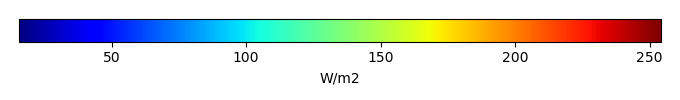MODEL MEANBIAS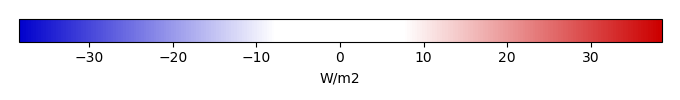BIAS SCORERMSE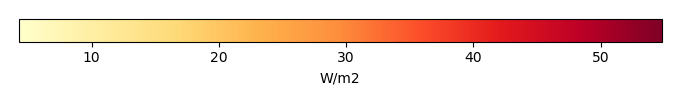RMSE SCOREBENCHMARK INTERANNUAL VARIABILITY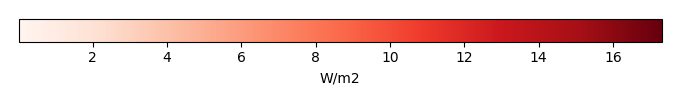MODEL INTERANNUAL VARIABILITYINTERANNUAL VARIABILITY SCOREBENCHMARK MAX MONTHMODEL MAX MONTHDIFFERENCE IN MAX MONTH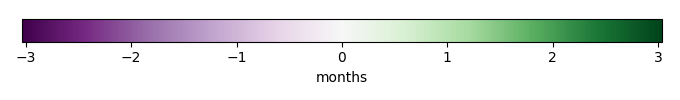SEASONAL CYCLE SCORESPATIAL TAYLOR DIAGRAMMODEL COLORS# Spatially integrated regional mean

MODEL COLORSREGIONAL MEANANNUAL CYCLEMONTHLY ANOMALYANNUAL CYCLE# All Models

BenchmarkCLM4CLM4.5CLM5# Data Information

creation_date: Thu May 8 23:03:44 PDT 2014

source_file: This product is generated from monthly 1 degree CERES EBAF Radiation observations